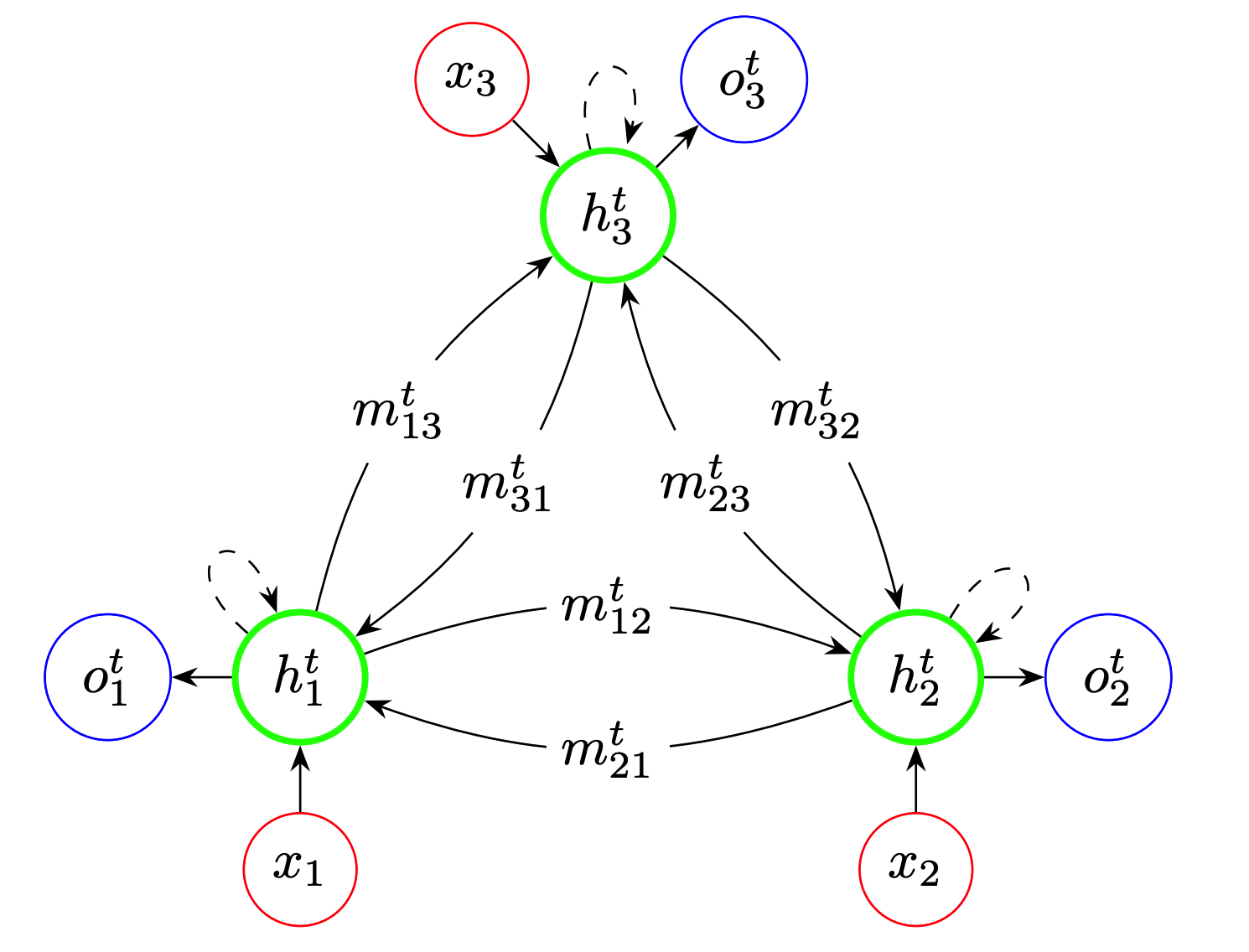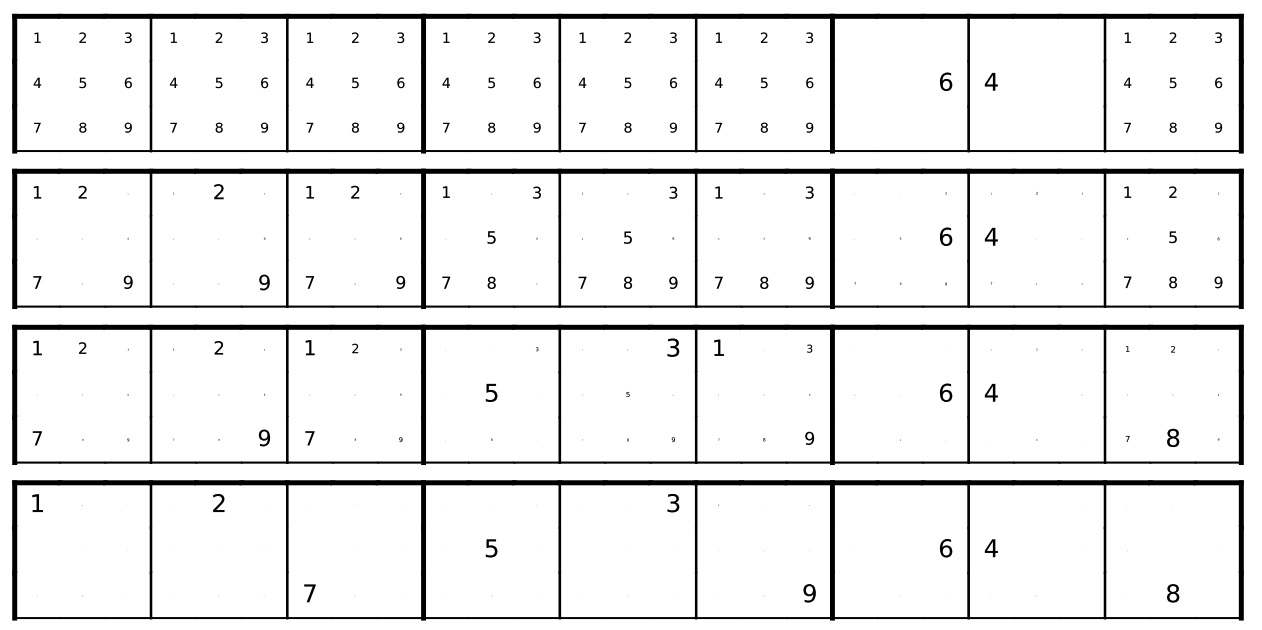# Recurrent Relational Networks

## WHY?

Some tasks such as Sudoku require serial steps of relational inference.

## WHAT?Recurrent relational network operates on a graph representation of objects. Message passing method is used to pass the relational information to neighbor nodes to solve the task. The loss is minimized at every step.

m_{ij}^t = f(h_i^{t-1}, h_j^{t-1})\\
m_j^t = \sum_{i\in N(j)}m^t_{ij}\\
h_j^t = g(h_j^{t-1}, x_j, m_j^t)\\
o_i^t = r(h_i^t)\\
l^t = -\sum_{i=1}^I \log o_i^t[y_i]

## So?This module showed stablly better performance in bAbI task. RRN showed good performance in the newly defined Pretty-CLEVER task. This paper also showed this network solving Sudoku well.

## Critic

It is clever to use message passing algorithm to solve relational tasks, but I think traing data is the issue(requires sequential answers for the task).

Powered by aiden